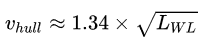# A Boat Displacement Speed and Boat Speed Calculation#Boat and Hull Speed Calculator

To calculate the speed of boat and calculate the boat hull speed with more accuracy.

Nau. Miles
Mins

## Formula

Boat Speed = ( DT / TT / 60 )

Where,

DT is Distance Travelled

TT is Time Travelledfeet

### Displacement Speed (or) Hull Speed Formula

Hull speed or displacement speed is the speed at which the wavelength of a vessels bow wave is equal to the waterline length of the vessel.Where,

LWL is is the length of the waterline in feet

vhull is the hull speed of the vessel in knots##Math Formulas##Age Calculators ►

Horse Age Calculator , Cow Age Calculator , Cat Age Calculator , Dog Age Calculator, Human Age Calculator 100 Days Old Calculator Date Difference Calculator